Home » TOTALYTD Function DAX

# TOTALYTD Function DAXTOTALYTD is a Power BI time intelligence function in DAX, which evaluates the year-to-date value of the expression in the current context.

DAX SYNTAX

`TOTALYTD(<expression>,<dates>[,<filter>][,<year_end_date>])`

expression An expression that returns a scalar value.

dates Is a column that contains dates.

The dates argument can be any of the following:

• A reference to a date/time column.
• A table expression that returns a single column of date/time values.
• A Boolean expression that defines a single-column table of date/time values.

filter Optional. An expression that specifies a filter to apply to the current context.

year_end_date Optional. Is a literal string with a date that defines the year-end date. Value for year_end_date can be specified as “5/15”, “May 15”, “15 May”, or any string that resolves to a month/day, Year portion is not required and is ignored. Default is December 31st.

Lets look at an example of using TOTALYTD DAX in Power BI.

Here we have a two datasets Dates and Financials , and there is a relationship exists between these two datasets on date fields as shown below.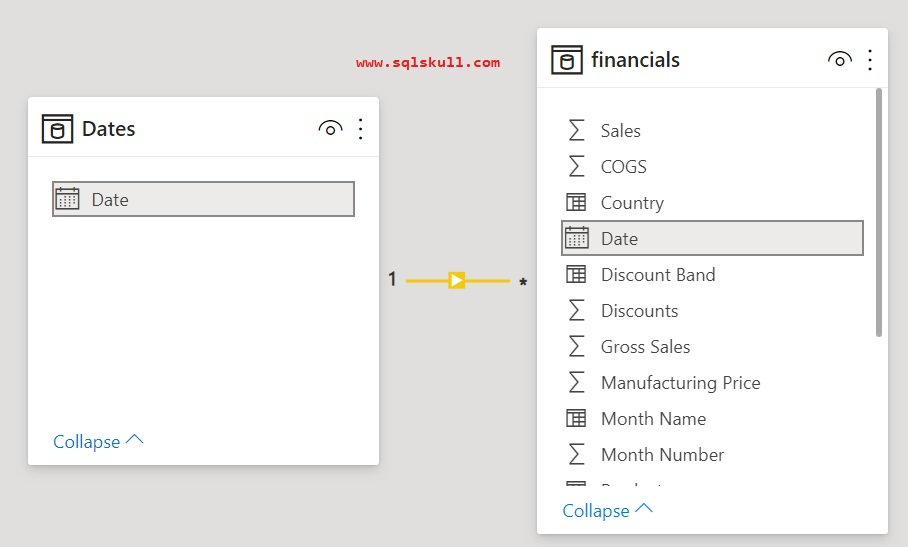Now we will create a simple sales report using these datasets which displays the Total Sales by Month and Year.

Lets take a Year and Months fields from Dates Dataset and Sales  from Financials dataset, now you can see we have a report which displays a Total Sales over Month and Year.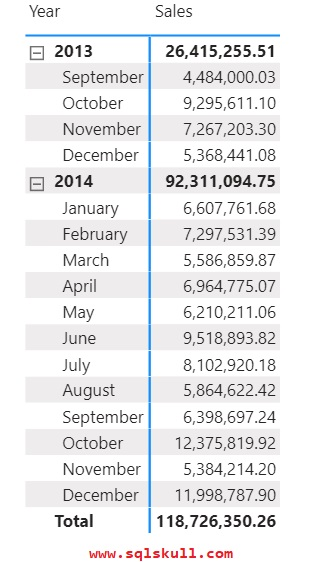Assume that, you want to display a cumulative or running subtotals in a year, then you can use TOTALYTD DAX function.

Lets create a measure named TOTALYTD_Sales that uses the TOTALYTD function which evaluates the year-to-date value of the Sales in the current context and returns the cumulative subtotals of sales in a year.

```TOTALYTD_Sales =
TOTALYTD ( SUM ( financials[ Sales] ), ( Dates[Date] ) )```After creating a DAX, lets drag the TOTALYTD_Sales measure into the report to see the output.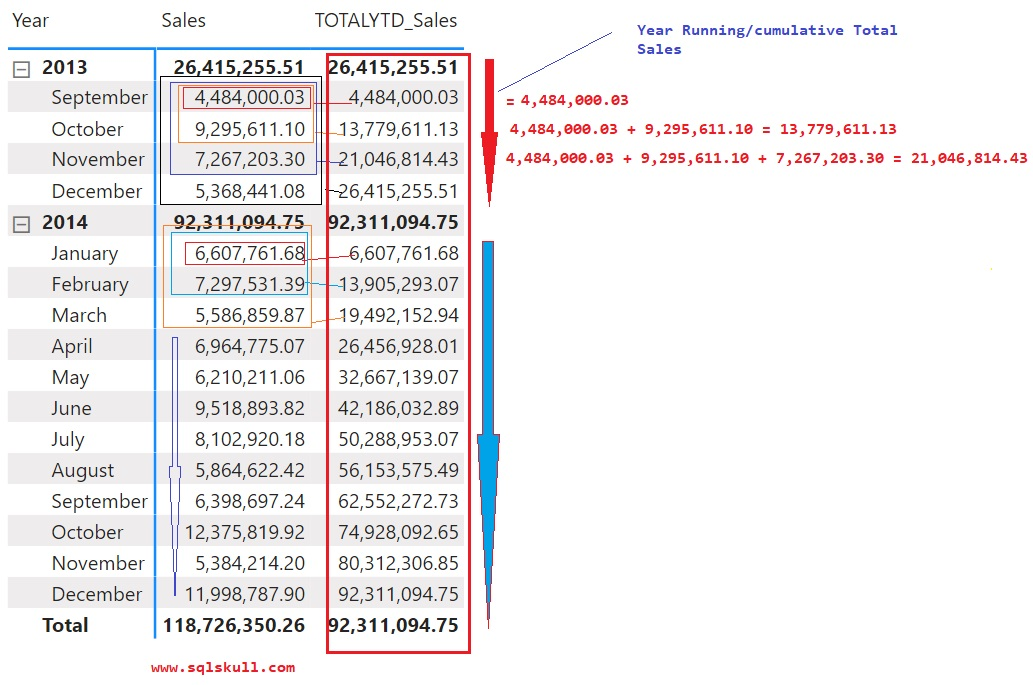You can see, it returns the “Year running total” for Sales.

Using filter with TOTALYTD

Lets see what else we can do with TOTALYTD DAX, assume that you want to see the Sales data for country Germany only.

Then you can also specify a third argument to TOTALYTD function that is filter to limit the sales data as shown below.

```TOTALYTD_Sales_Ger =
TOTALYTD (SUM ( financials[ Sales] ),( Dates[Date] ),financials[Country]="Germany" )```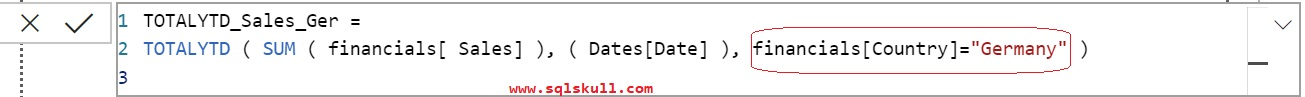Lets create one more DAX which returns the sales data for country Germany only, so that we can validate the output of above DAX TOTALYTD_Sales_Ger.

`Sales_Germany = CALCULATE(SUM(financials[ Sales]), financials[Country]="Germany")`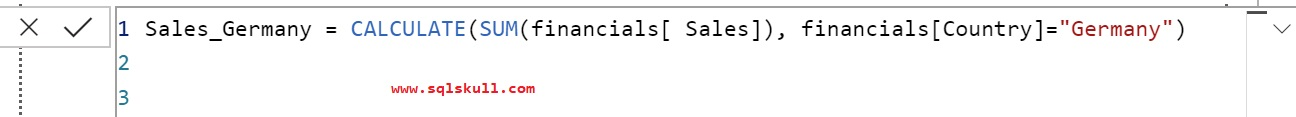After creating a DAX, lets drag the Sales_Germany and TOTALYTD_Sales_Ger into report to see the output.You can see, it returns “Year running total” for Sales in country Germany.

#### Specifying a fiscal year end date to TOTALYTD

So far we have seen, the TOTALYTD returns a year running total and the fiscal year end on December 31st (default).

Assume that, you want to see the total YTD sales for a fiscal year ending on Mar 31st, then you can specify the year_end_date value “3/31” that is month/day.

Lets modifying above DAX as shown below.

So far, we have a YTD for fiscal year ending on December 31st (Default), now we will change it to march 31st, lets take a fresh report to avoid any confusion, you can see below report that display a over all total Sales, and Total Sales for country Germany by month and year.Lets change the fiscal year to March 31st, you need to specify a fourth argument value as “3/31” in TOTALYTD DAX as shown below.

```TOTALYTD_Sales_Ger_FY_31mar =
TOTALYTD (
SUM ( financials[ Sales] ),
( Dates[Date] ),
financials[Country] = "Germany",
"3/31"
)```After creating a DAX, lets drag the DAX TOTALYTD_Sales_Ger_FY_31mar in report to see the output.You can see, it returns a “Year running total” for sales and you can see now fiscal year ending on March 31st instead of December 31st.